### IMO Shortlist 2008 problem N2

Kvaliteta:
Avg: 0,0
Težina:
Avg: 6,0
Let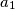$a_1$,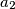$a_2$,$\ldots$,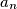$a_n$ be distinct positive integers,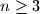$n\ge 3$. Prove that there exist distinct indices$i$ and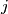$j$ such that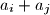$a_i + a_j$ does not divide any of the numbers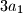$3a_1$,$3a_2$,$\ldots$,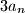$3a_n$.

Proposed by Mohsen Jamaali, Iran
Izvor: Međunarodna matematička olimpijada, shortlist 2008